• pytorch tensor的统计属性1. 默认类型2. 范数3. max, min, argmin, argmax3.1 max 函数3.2 min 函数3.3 argmin 函数3.4 argmax 函数4. mean, sum4.1 mean 函数4.2 sum 函数5. prod5.1 prod 函数6. kthvalue, topk6.1...


pytorch tensor的统计属性
1. 默认类型2. 范数3. max, min, argmin, argmax3.1 max 函数3.2 min 函数3.3 argmin 函数3.4 argmax 函数
4. mean, sum4.1 mean 函数4.2 sum 函数
5. prod5.1 prod 函数
6. kthvalue, topk6.1 kthvalue 函数6.2 topk 函数

1. 默认类型
torch.set_default_tensor_type(t)：设置

p

y

t

o

r

c

h

pytorch

中默认的浮点类型。
数据类型CPU TensorGPU Tensor16 bit 单精度浮点无torch.cuda.HalfTensor32 bit 浮点torch.FloatTensortorch.cuda.FloatTensor64 bit 浮点torch.DoubleTensortorch.cuda.DoubleTensor
2. 范数

0

−

范

数

0-范数

：非零元素的个数。

1

−

范

数

1-范数

：

∣

∣

x

∣

∣

1

=

∣

x

1

∣

+

∣

x

2

∣

+

∣

x

3

∣

+

⋯

+

∣

x

n

∣

||x||_1=|x_1|+|x_2|+|x_3|+\cdots+|x_n|

（向量元素绝对值之和），

x

x

到原点的曼哈顿距离。

向

量

2

−

范

数

向量\ 2-范数

：

∣

∣

x

∣

∣

2

=

∣

x

1

∣

2

+

∣

x

2

∣

2

+

∣

x

3

∣

2

+

⋯

+

∣

x

n

∣

2

2

||x||_2=\sqrt{|x_1|^2+|x_2|^2+|x_3|^2+\cdots+|x_n|^2}

，

2

2

-范数也称为 *

E

u

c

l

i

d

Euclid

范数（欧几里得范数，常用计算向量长度），即向量元素绝对值的平方和再开方，表示

x

x

到零点的欧式距离。

矩

阵

F

−

范

数

（

参

数

f

r

o

）

矩阵\ F-范数（参数\ fro）

：

∣

∣

A

∣

∣

F

=

(

∑

i

=

1

m

∑

j

=

1

m

a

i

,

j

2

)

1

2

\displaystyle ||A||_F=(\sum^{m}_{i=1}\sum^{m}_{j=1}{a^2_{i,j}})^{\frac{1}{2}}

，称为Frobenius范数，即矩阵元素绝对值的平方和再开平方。

p

−

范

数

p-范数

：

∣

∣

x

∣

∣

p

=

∣

x

1

∣

p

+

∣

x

2

∣

p

+

∣

x

3

∣

p

+

⋯

+

∣

x

n

∣

p

p

||x||_p=\sqrt[p]{|x_1|^p+|x_2|^p+|x_3|^p+\cdots+|x_n|^p}

（

p

p

指的是求

p

p

范数的

p

p

值）。

∞

−

范

数

（

参

数

i

n

f

）

\infty-范数（参数\ inf）

：

∣

∣

x

∣

∣

∞

=

m

a

x

∣

x

i

∣

||x||_\infty=max|x_i|

，即所有向量元素绝对值中的最大值。

−

∞

−

范

数

（

参

数

−

i

n

f

）

-\infty-范数（参数\ -inf）

：

∣

∣

x

∣

∣

−

∞

=

m

i

n

∣

x

i

∣

||x||_{-\infty}=min|x_i|

，即所有向量元素绝对值中的最小值。
torch.norm(input, p='fro', dim=None, keepdim=False, out=None, dtype=None)：
input：输入

t

e

n

s

o

r

tensor

。p(int, float, inf, -inf, 'fro', 'nuc', optional)：范数计算中的幂指数值，默认为’fro’。dim (int，2-tuple，2-list， optional)：指定计算的维度。
如果是一个整数值，向量范数将被计算；如果是一个大小为

2

2

的元组，矩阵范数将被计算；如果为

N

o

n

e

None

，当输入

t

e

n

s

o

r

tensor

只有二维时计算矩阵范数；当输入只有一维时则计算向量范数。如果输入

t

e

n

s

o

r

tensor

超过

2

2

维，向量范数将被应用在最后一维。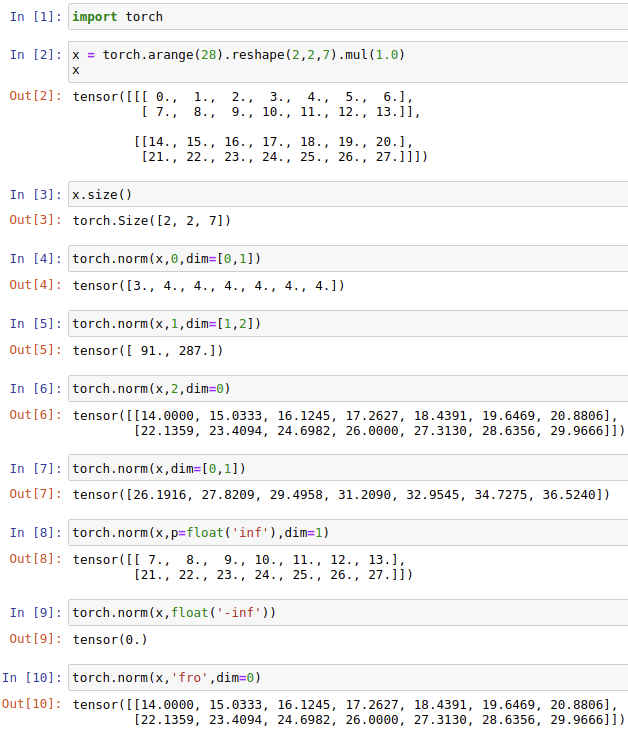3. max, min, argmin, argmax
3.1 max 函数
torch.max(input, dim, keepdim)：返回输入张量

i

n

p

u

t

input

在给定维度

d

i

m

dim

上的最大值，并同时返回每个最大值的位置索引。

k

e

e

p

d

i

m

keepdim

表示是否需要保持输出的维度与输入一样，

k

e

e

p

d

i

m

=

T

r

u

e

keepdim=True

表示输出和输入的维度一样，

k

e

e

p

d

i

m

=

F

a

l

s

e

keepdim=False

（默认）表示输出的维度被压缩了，也就是输出会比输入低一个维度。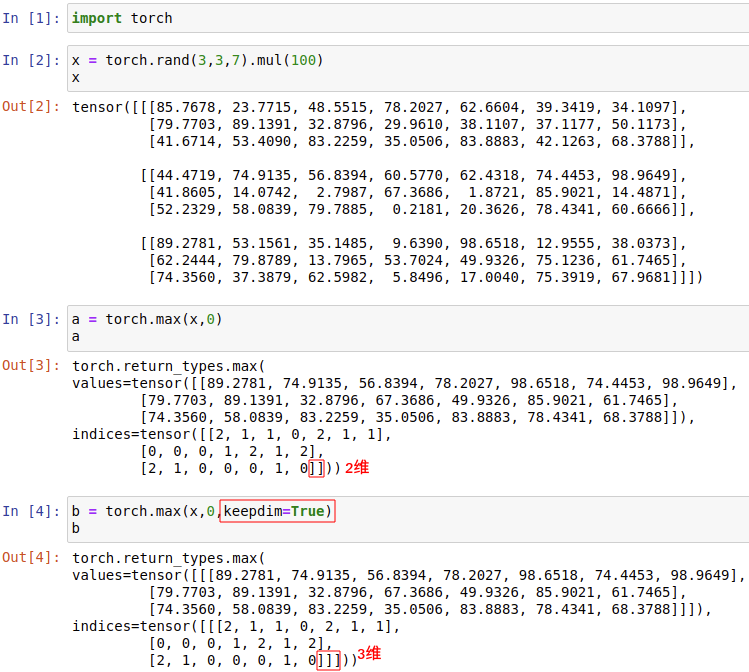3.2 min 函数
torch.min(input, dim, keepdim)：返回输入张量

i

n

p

u

t

input

在给定维度

d

i

m

dim

上的最小值，并同时返回每个最小值的位置索引。

k

e

e

p

d

i

m

keepdim

表示是否需要保持输出的维度与输入一样，

k

e

e

p

d

i

m

=

T

r

u

e

keepdim=True

表示输出和输入的维度一样，

k

e

e

p

d

i

m

=

F

a

l

s

e

keepdim=False

（默认）表示输出的维度被压缩了，也就是输出会比输入低一个维度。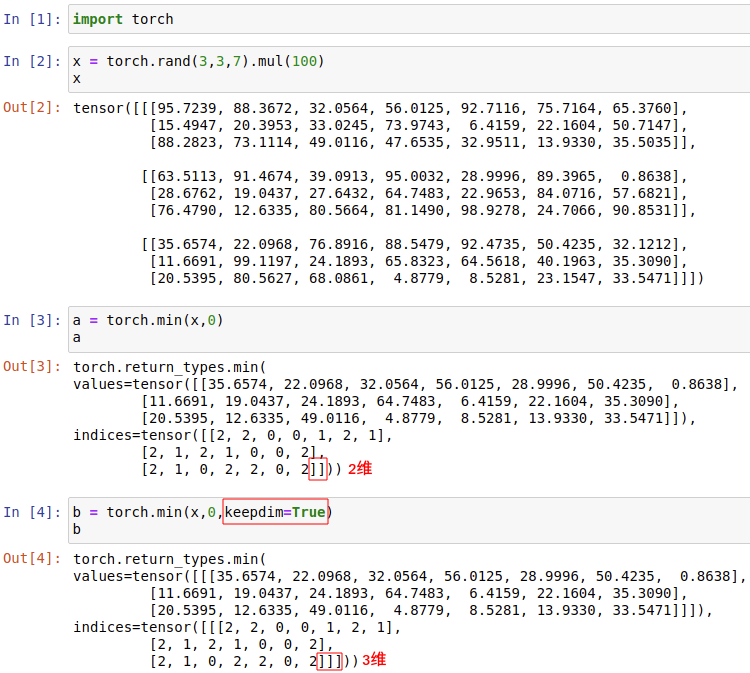3.3 argmin 函数
torch.argmin(input, dim, keepdim)：返回输入张量

i

n

p

u

t

input

在给定维度

d

i

m

dim

上最小值的位置索引。

k

e

e

p

d

i

m

keepdim

表示是否需要保持输出的维度与输入一样，

k

e

e

p

d

i

m

=

T

r

u

e

keepdim=True

表示输出和输入的维度一样，

k

e

e

p

d

i

m

=

F

a

l

s

e

keepdim=False

（默认）表示输出的维度被压缩了，也就是输出会比输入低一个维度。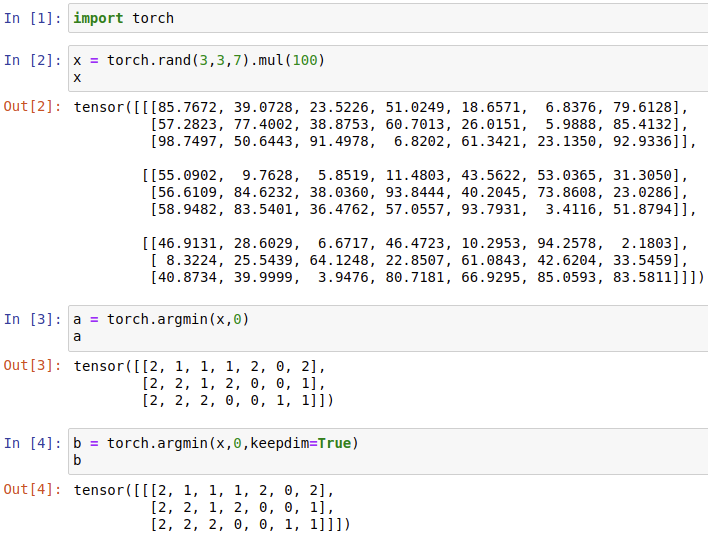3.4 argmax 函数
torch.argmax(input, dim, keepdim)：返回输入张量

i

n

p

u

t

input

在给定维度

d

i

m

dim

上最大值的位置索引。

k

e

e

p

d

i

m

keepdim

表示是否需要保持输出的维度与输入一样，

k

e

e

p

d

i

m

=

T

r

u

e

keepdim=True

表示输出和输入的维度一样，

k

e

e

p

d

i

m

=

F

a

l

s

e

keepdim=False

（默认）表示输出的维度被压缩了，也就是输出会比输入低一个维度。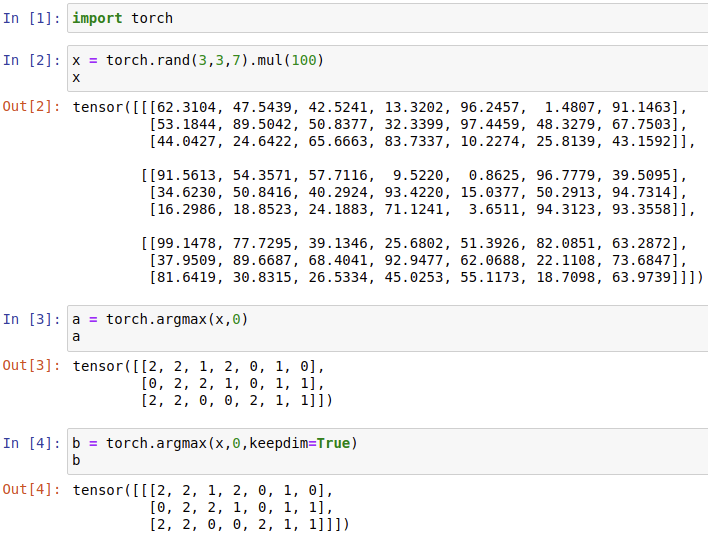4. mean, sum
4.1 mean 函数
torch.mean(input, dim, keepdim)：返回输入张量

i

n

p

u

t

input

在给定维度

d

i

m

dim

上的平均值，并同时返回每个平均值的位置索引。

k

e

e

p

d

i

m

keepdim

表示是否需要保持输出的维度与输入一样，

k

e

e

p

d

i

m

=

T

r

u

e

keepdim=True

表示输出和输入的维度一样，

k

e

e

p

d

i

m

=

F

a

l

s

e

keepdim=False

（默认）表示输出的维度被压缩了，也就是输出会比输入低一个维度。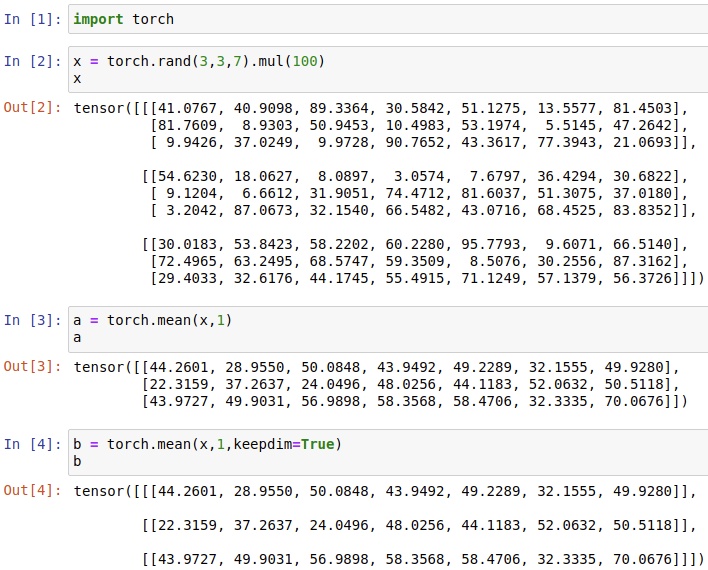4.2 sum 函数
torch.sum(input, dim, keepdim)：返回输入张量

i

n

p

u

t

input

在给定维度

d

i

m

dim

上的总和，并同时返回每个总和的位置索引。

k

e

e

p

d

i

m

keepdim

表示是否需要保持输出的维度与输入一样，

k

e

e

p

d

i

m

=

T

r

u

e

keepdim=True

表示输出和输入的维度一样，

k

e

e

p

d

i

m

=

F

a

l

s

e

keepdim=False

（默认）表示输出的维度被压缩了，也就是输出会比输入低一个维度。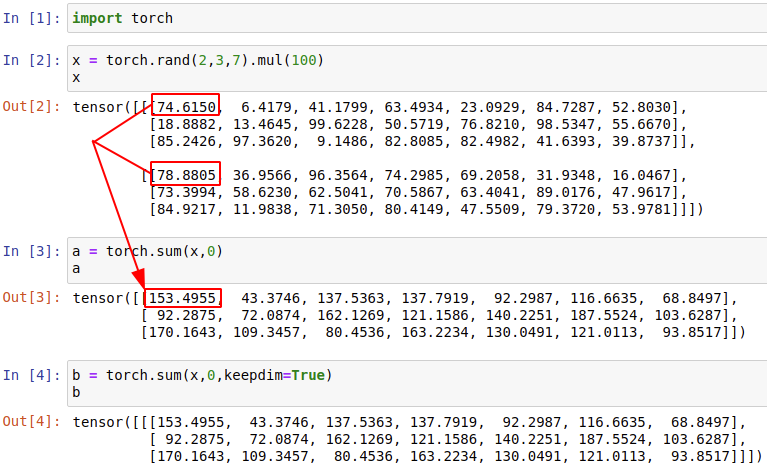5. prod
5.1 prod 函数
torch.prod(input, dim, keepdim)：返回输入张量

i

n

p

u

t

input

在给定维度

d

i

m

dim

上所有元素的乘积，并同时返回每个乘积的位置索引。

k

e

e

p

d

i

m

keepdim

表示是否需要保持输出的维度与输入一样，

k

e

e

p

d

i

m

=

T

r

u

e

keepdim=True

表示输出和输入的维度一样，

k

e

e

p

d

i

m

=

F

a

l

s

e

keepdim=False

（默认）表示输出的维度被压缩了，也就是输出会比输入低一个维度。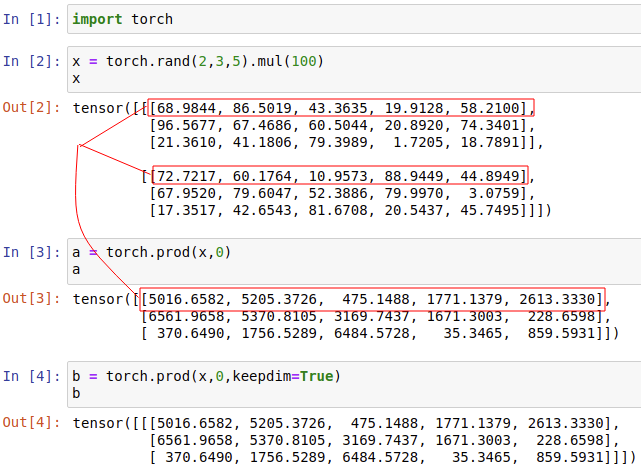6. kthvalue, topk
6.1 kthvalue 函数
torch.kthvalue(input, k, dim=None, out=None)：取输入张量

i

n

p

u

t

input

指定维度上第

k

k

个最小值，若不指定

d

i

m

dim

，则默认为

i

n

p

u

t

input

的最后一维。返回一个元组，其中

i

n

d

i

c

e

s

indices

是原始输入张量

i

n

p

u

t

input

中沿

d

i

m

dim

维的第

k

k

个最小值下标。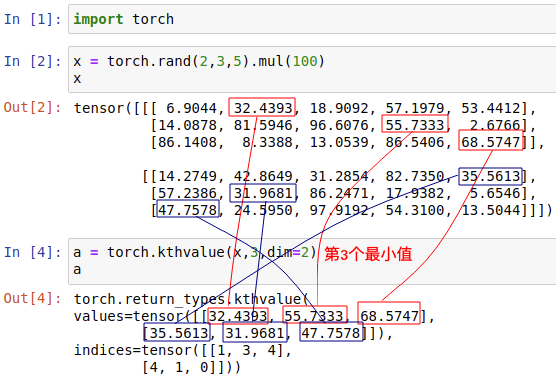6.2 topk 函数
torch.topk(input, k, dim=None, largest=True, sorted=True, out=None)：沿给定

d

i

m

dim

维度返回输入张量

i

n

p

u

t

input

中

k

k

个最大值，不指定

d

i

m

dim

，则默认为最后一维，如果

l

a

r

g

e

s

t

largest

为

F

a

l

s

e

False

，则返回最小的

k

k

个值。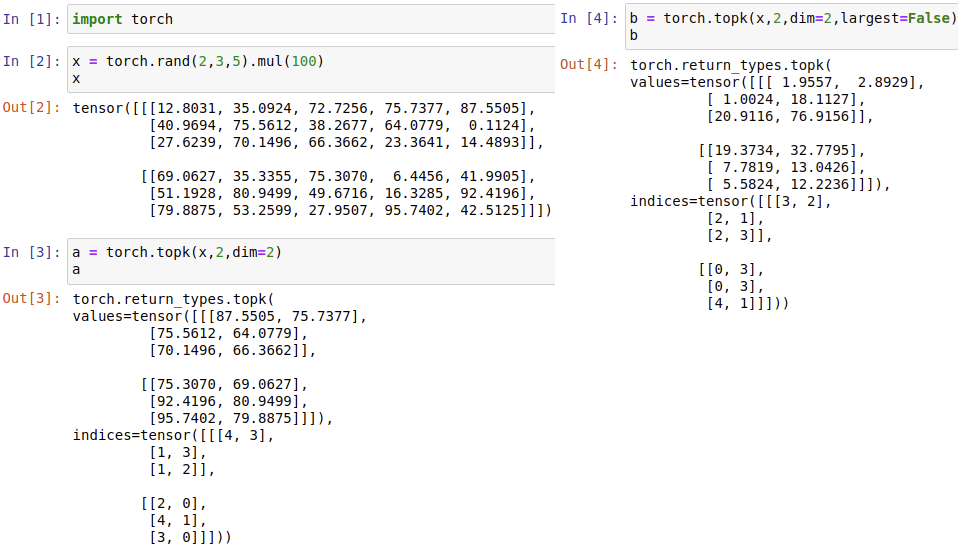展开全文深度学习 神经网络
• ## pytorchTensor及其基本操作

万次阅读 多人点赞 2019-04-04 09:05:53
由于之前的草稿都没了，现在只有重写…. 我好痛苦本章只是对pytorch的常规操作进行一个总结，大家看过有脑子里有印象就好，知道有...本章导视图 Tensor attributes:在tensor attributes中有三个类，分别为torch.d...
转自： https://zhuanlan.zhihu.com/p/36233589

由于之前的草稿都没了，现在只有重写…. 我好痛苦
本章只是对pytorch的常规操作进行一个总结，大家看过有脑子里有印象就好，知道有这么个东西，需要的时候可以再去详细的看，另外也还是需要在实战中多运用。

本章导视图

Tensor attributes:
在tensor attributes中有三个类，分别为torch.dtype, torch.device, 和 torch.layout
其中， torch.dtype 是展示 torch.Tensor 数据类型的类，pytorch 有八个不同的数据类型,下表是完整的 dtype 列表.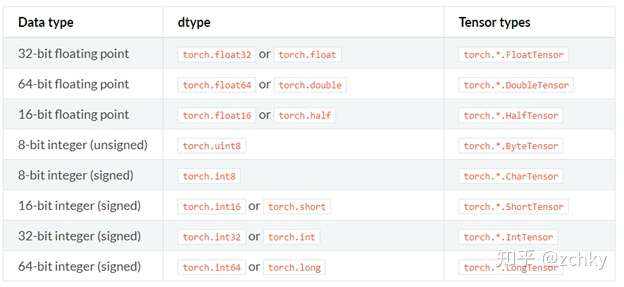Torch.device 是表现 torch.Tensor被分配的设备类型的类，其中分为’cpu’ 和 ‘cuda’两种，如果设备序号没有显示则表示此 tensor 被分配到当前设备, 比如: 'cuda' 等同于 'cuda': X , X 为torch.cuda.current _device() 返回值
我们可以通过 tensor.device 来获取其属性，同时可以利用字符或字符+序号的方式来分配设备

通过字符串：
>>> torch.device('cuda:0')
device(type='cuda', index=0)
>>> torch.device('cpu')
device(type='cpu')
>>> torch.device('cuda') # 当前设备
device(type='cuda')


通过字符串和设备序号： >>> torch.device(‘cuda’, 0) device(type=‘cuda’, index=0) >>> torch.device(‘cpu’, 0) device(type=‘cpu’, index=0)
此外，cpu 和 cuda 设备的转换使用 ‘to’ 来实现：

>>> device_cpu = torch.device(“cuda”)  #声明cuda设备
>>> device_cuda = torch.device(‘cuda’)  #设备cpu设备
>>> data = torch.Tensor()
>>> data.to(device_cpu)  #将数据转为cpu格式
>>> data.to(device_cuda)   #将数据转为cuda格式


torch.layout 是表现 torch.Tensor 内存分布的类，目前只支持 torch.strided

创建tensor
直接创建
data - 可以是list, tuple, numpy array, scalar或其他类型
dtype - 可以返回想要的tensor类型
device - 可以指定返回的设备
需要注意的是，torch.tensor 总是会复制 data, 如果你想避免复制，可以使 torch.Tensor. detach()，如果是从 numpy 中获得数据，那么你可以用 torch.from_numpy(), 注from_numpy() 是共享内存的

>>> torch.tensor([[0.1, 1.2], [2.2, 3.1], [4.9, 5.2]])
tensor([[ 0.1000,  1.2000],
[ 2.2000,  3.1000],
[ 4.9000,  5.2000]])


>>> torch.tensor([0, 1]) # Type inference on data tensor([ 0, 1])
>>> torch.tensor([[0.11111, 0.222222, 0.3333333]], dtype=torch.float64, device=torch.device(‘cuda:0’)) # creates a torch.cuda.DoubleTensor tensor([[ 0.1111, 0.2222, 0.3333]], dtype=torch.float64, device=‘cuda:0’)
>>> torch.tensor(3.14159) # Create a scalar (zero-dimensional tensor) tensor(3.1416)
>>> torch.tensor([]) # Create an empty tensor (of size (0,)) tensor([])

从numpy中获得数据
torch.from_numpy(ndarry)
注：生成返回的tensor会和ndarry共享数据，任何对tensor的操作都会影响到ndarry,反之亦然

>>> a = numpy.array([1, 2, 3])
>>> t = torch.from_numpy(a)
>>> t
tensor([ 1,  2,  3])
>>> t = -1
>>> a
array([-1,  2,  3])


创建特定的tensor
根据数值要求：

torch.zeros(*sizes, out=None, …)# 返回大小为sizes的零矩阵


torch.zeros_like(input, …) # 返回与input相同size的零矩阵
torch.ones(*sizes, out=None, …) #f返回大小为sizes的单位矩阵
torch.ones_like(input, …) #返回与input相同size的单位矩阵
torch.full(size, fill_value, …) #返回大小为sizes,单位值为fill_value的矩阵
torch.full_like(input, fill_value, …) 返回与input相同size，单位值为fill_value的矩阵
torch.arange(start=0, end, step=1, …) #返回从start到end, 单位步长为step的1-d tensor.
torch.linspace(start, end, steps=100, …) #返回从start到end, 间隔中的插值数目为steps的1-d tensor
torch.logspace(start, end, steps=100, …) #返回1-d tensor ，从10start到10end的steps个对数间隔
根据矩阵要求:

torch.eye(n, m=None, out=None,…) #返回2-D 的单位对角矩阵


torch.empty(*sizes, out=None, …) #返回被未初始化的数值填充，大小为sizes的tensor
torch.empty_like(input, …) # 返回与input相同size,并被未初始化的数值填充的tensor

随机采用生成:

torch.normal(mean, std, out=None)


torch.rand(*size, out=None, dtype=None, …) #返回[0,1]之间均匀分布的随机数值
torch.rand_like(input, dtype=None, …) #返回与input相同size的tensor, 填充均匀分布的随机数值
torch.randint(low=0, high, size,…) #返回均匀分布的[low,high]之间的整数随机值
torch.randint_like(input, low=0, high, dtype=None, …) #
torch.randn(*sizes, out=None, …) #返回大小为size,由均值为0，方差为1的正态分布的随机数值
torch.randn_like(input, dtype=None, …)
torch.randperm(n, out=None, dtype=torch.int64) # 返回0到n-1的数列的随机排列

操作tensor
基本操作：
Joining ops:

torch.cat(seq,dim=0,out=None) # 沿着dim连接seq中的tensor, 所有的tensor必须有相同的size或为empty， 其相反的操作为 torch.split() 和torch.chunk()
torch.stack(seq, dim=0, out=None) #同上


#注: .cat 和 .stack的区别在于 cat会增加现有维度的值,可以理解为续接，stack会新加增加一个维度，可以 理解为叠加 >>> a=torch.Tensor([1,2,3]) >>> torch.stack((a,a)).size() torch.size(2,3) >>> torch.cat((a,a)).size() torch.size(6)

torch.gather(input, dim, index, out=None) #返回沿着dim收集的新的tensor
>> t = torch.Tensor([[1,2],[3,4]])
>> index = torch.LongTensor([[0,0],[1,0]])
>> torch.gather(t, 0, index) #由于 dim=0,所以结果为
| t[index[0, 0] 0]   t[index[0, 1] 1] |
| t[index[1, 0] 0]   t[index[1, 1] 1] |


对于3-D 的张量来说，可以作为
out[i][j][k] = input[index[i][j][k]][j][k] # if dim == 0 out[i][j][k] = input[i][index[i][j][k]][k] # if dim == 1 out[i][j][k] = input[i][j][index[i][j][k]] # if dim == 2

clicing ops:

torch.split(tensor, split_size_or_sections, dim=0) #将tensor 拆分成相应的组块
torch.chunk(tensor, chunks, dim=0) #将tensor 拆分成相应的组块， 最后一块会小一些如果不能整除的话#


#注：split和chunk的区别在于： split的split_size_or_sections 表示每一个组块中的数据大小，chunks表示组块的数量 >>> a = torch.Tensor([1,2,3]) >>> torch.split(a,1) (tensor([1.]), tensor([2.]), tensor([3.])) >>> torch.chunk(a,1) (tensor([ 1., 2., 3.]),)

Indexing ops:

torch.index_select(input, dim, index, out=None) #返回沿着dim的指定tensor, index需为longTensor类型，不共用内存


torch.masked_select(input, mask, out=None) #根据mask来返回input的值其为1-D tensor. Mask为ByteTensor, true返回，false不返回，返回值不共用内存 >>> x = torch.randn(3, 4) >>> x tensor([[ 0.3552, -2.3825, -0.8297, 0.3477], [-1.2035, 1.2252, 0.5002, 0.6248], [ 0.1307, -2.0608, 0.1244, 2.0139]]) >>> mask = x.ge(0.5) >>> mask tensor([[ 0, 0, 0, 0], [ 0, 1, 1, 1], [ 0, 0, 0, 1]], dtype=torch.uint8) >>> torch.masked_select(x, mask) tensor([ 1.2252, 0.5002, 0.6248, 2.0139])

Mutation ops:

torch.transpose(input, dim0, dim1, out=None) #返回dim0和dim1交换后的tensor
torch.t(input, out=None) #专为2D矩阵的转置，是transpose的便捷函数


torch.squeeze(input, dim, out=None) #默认移除所有size为1的维度，当dim指定时，移除指定size为1的维度. 返回的tensor会和input共享存储空间，所以任何一个的改变都会影响另一个 torch.unsqueeze(input, dim, out=None) #扩展input的size, 如 A x B 变为 1 x A x B
torch.reshape(input, shape) #返回size为shape具有相同数值的tensor, 注意 shape=(-1,)这种表述，-1表示任意的。 #注 reshape(-1,) >>> a=torch.Tensor([1,2,3,4,5]) #a.size 是 torch.size(5) >>> b=a.reshape(1,-1) #表示第一维度是1，第二维度按a的size填充满 >>> b.size() torch.size([1,5])
torch.where(condition,x,y) #根据condition的值来相应x,y的值，true返回x的值，false返回y的值，形成新的tensor
torch.unbind(tensor, dim=0) #返回tuple 解除指定的dim的绑定,相当于按指定dim拆分 >>> a=torch.Tensor([[1,2,3],[2,3,4]]) >>> torch.unbind(a,dim=0) (torch([1,2,3]),torch([2,3,4])) # 将一个(2,3) 分为两个(3)
torch.nonzero(input, out=None) # 返回非零值的索引， 每一行都是一个非零值的索引值 >>> torch.nonzero(torch.tensor([1, 1, 1, 0, 1])) tensor([[ 0], [ 1], [ 2], [ 4]]) >>> torch.nonzero(torch.tensor([[0.6, 0.0, 0.0, 0.0], [0.0, 0.4, 0.0, 0.0], [0.0, 0.0, 1.2, 0.0], [0.0, 0.0, 0.0,-0.4]])) tensor([[ 0, 0], [ 1, 1], [ 2, 2], [ 3, 3]])

Tensor操作
点对点操作
三角函数：

torch.abs(input, out=None)
torch.acos(input, out=None)
torch.asin(input, out=None)
torch.atan(input, out=None)
torch.atan2(input, inpu2, out=None)
torch.cos(input, out=None)
torch.cosh(input, out=None)
torch.sin(input, out=None)
torch.sinh(input, out=None)
torch.tan(input, out=None)
torch.tanh(input, out=None)


基本运算，加减乘除

Torch.add(input, value, out=None)
torch.div(input, value, out=None)
.div(input, other, out=None)
torch.mul(input, value, out=None)
.mul(input, other, out=None)


对数运算：

torch.log(input, out=None)  # y_i=log_e(x_i)
torch.log1p(input, out=None)  #y_i=log_e(x_i+1)
torch.log2(input, out=None)   #y_i=log_2(x_i)
torch.log10(input,out=None)  #y_i=log_10(x_i)


幂函数：

torch.pow(input, exponent, out=None)  # y_i=input^(exponent)


指数运算

torch.exp(tensor, out=None)    #y_i=e^(x_i)
torch.expm1(tensor, out=None)   #y_i=e^(x_i) -1


截断函数

torch.ceil(input, out=None)   #返回向正方向取得最小整数
torch.floor(input, out=None)  #返回向负方向取得最大整数


torch.round(input, out=None) #返回相邻最近的整数，四舍五入
torch.trunc(input, out=None) #返回整数部分数值 torch.frac(tensor, out=None) #返回小数部分数值
torch.fmod(input, divisor, out=None) #返回input/divisor的余数 torch.remainder(input, divisor, out=None) #同上

其他运算

torch.erf(tensor， out=None)


torch.erfinv(tensor, out=None)
torch.sigmoid(input, out=None)
torch.clamp(input, min, max out=None) #返回 input<min,则返回min, input>max,则返回max,其余返回input
torch.neg(input, out=None) #out_i=-1*(input)
torch.reciprocal(input, out=None) # out_i= 1/input_i
torch.sqrt(input, out=None) # out_i=sqrt(input_i) torch.rsqrt(input, out=None) #out_i=1/(sqrt(input_i))
torch.sign(input, out=None) #out_i=sin(input_i) 大于0为1，小于0为-1
torch.lerp(start, end, weight, out=None)

降维操作

torch.argmax(input, dim=None, keepdim=False) #返回最大值排序的索引值
torch.argmin(input, dim=None, keepdim=False)  #返回最小值排序的索引值


torch.cumprod(input, dim, out=None) #y_i=x_1 * x_2 * x_3 … x_i torch.cumsum(input, dim, out=None) #y_i=x_1 + x_2 + … + x_i
torch.dist(input, out, p=2) #返回input和out的p式距离 torch.mean() #返回平均值 torch.sum() #返回总和 torch.median(input) #返回中间值 torch.mode(input) #返回众数值 torch.unique(input, sorted=False) #返回1-D的唯一的tensor,每个数值返回一次. >>> output = torch.unique(torch.tensor([1, 3, 2, 3], dtype=torch.long)) >>> output tensor([ 2, 3, 1])
torch.std( #返回标准差) torch.var() #返回方差
torch.norm(input, p=2) #返回p-norm的范式 torch.prod(input, dim, keepdim=False) #返回指定维度每一行的乘积

对比操作：

torch.eq(input, other, out=None)  #按成员进行等式操作，相同返回1
torch.equal(tensor1, tensor2) #如果tensor1和tensor2有相同的size和elements，则为true
>>> torch.eq(torch.tensor([[1, 2], [3, 4]]), torch.tensor([[1, 1], [4, 4]]))
tensor([[ 1,  0],
[ 0,  1]], dtype=torch.uint8)
>>> torch.eq(torch.tensor([[1, 2], [3, 4]]), torch.tensor([[1, 1], [4, 4]]))
tensor([[ 1,  0],
[ 0,  1]], dtype=torch.uint8)


torch.ge(input, other, out=None) # input>= other torch.gt(input, other, out=None) # input>other torch.le(input, other, out=None) # input=<other torch.lt(input, other, out=None) # input<other torch.ne(input, other, out=None) # input != other 不等于
torch.max() # 返回最大值 torch.min() # 返回最小值 torch.isnan(tensor) #判断是否为’nan’ torch.sort(input, dim=None, descending=False, out=None) #对目标input进行排序 torch.topk(input, k, dim=None, largest=True, sorted=True, out=None) #沿着指定维度返回最大k个数值及其索引值 torch.kthvalue(input, k, dim=None, deepdim=False, out=None) #沿着指定维度返回最小k个数值及其索引值

频谱操作

torch.fft(input, signal_ndim, normalized=False)
torch.ifft(input, signal_ndim, normalized=False)
torch.rfft(input, signal_ndim, normalized=False, onesided=True)
torch.irfft(input, signal_ndim, normalized=False, onesided=True)
torch.stft(signa, frame_length, hop, …)


其他操作：

torch.cross(input, other, dim=-1, out=None)  #叉乘(外积)


torch.dot(tensor1, tensor2) #返回tensor1和tensor2的点乘
torch.mm(mat1, mat2, out=None) #返回矩阵mat1和mat2的乘积
torch.eig(a, eigenvectors=False, out=None) #返回矩阵a的特征值/特征向量
torch.det(A) #返回矩阵A的行列式
torch.trace(input) #返回2-d 矩阵的迹(对对角元素求和)
torch.diag(input, diagonal=0, out=None) #
torch.histc(input, bins=100, min=0, max=0, out=None) #计算input的直方图
torch.tril(input, diagonal=0, out=None) #返回矩阵的下三角矩阵，其他为0
torch.triu(input, diagonal=0, out=None) #返回矩阵的上三角矩阵，其他为0

Tips:
获取python number:
由于pytorch 0.4后，python number的获取统一通过 .item()方式实现：

>>> a = torch.Tensor([1,2,3])
>>> a   #直接取索引返回的是tensor数据
tensor(1.)
>>> a.item()  #获取python number
1


tensor设置
判断:

torch.is_tensor()  #如果是pytorch的tensor类型返回true
torch.is_storage() # 如果是pytorch的storage类型返回ture


这里还有一个小技巧，如果需要判断tensor是否为空，可以如下

>>> a=torch.Tensor()
>>> len(a)
0
>>> len(a) is 0
True


设置: 通过一些内置函数，可以实现对tensor的精度, 类型，print打印参数等进行设置

torch.set_default_dtype(d)  #对torch.tensor() 设置默认的浮点类型


torch.set_default_tensor_type() # 同上，对torch.tensor()设置默认的tensor类型 >>> torch.tensor([1.2, 3]).dtype # initial default for floating point is torch.float32 torch.float32 >>> torch.set_default_dtype(torch.float64) >>> torch.tensor([1.2, 3]).dtype # a new floating point tensor torch.float64 >>> torch.set_default_tensor_type(torch.DoubleTensor) >>> torch.tensor([1.2, 3]).dtype # a new floating point tensor torch.float64
torch.get_default_dtype() #获得当前默认的浮点类型torch.dtype
torch.set_printoptions(precision=None, threshold=None, edgeitems=None, linewidth=None, profile=None）#)
设置printing的打印参数


展开全文• 2019独角兽企业重金招聘Python工程师标准>>> def TestTensor(): ...torch.max返回input张量中所有元素的最大值 torch.min返回input张量中所有元素的最小值 torch.sort按值按升序...


2019独角兽企业重金招聘Python工程师标准>>>def TestTensor():
x1 = torch.empty(5,3)#构建一个5*3张量或矩阵;注意这里是(5,3),而不是[5,3];其并未进行初始化;
x2 = torch.empty(5,3)#构建一个随机初始化的矩阵;
x3 = torch.zeros(5,3,dtype=torch.long)#构建矩阵使用0进行填充,并指定了填充类型;
x = torch.tensor([2,3])#直接从python列表构建矩阵;
x = x.new_ones(5,3,dtype=torch.double)#注意这里是x.new_ones,不是torch.new_ones;类型为double并且依据值为1张量的属性进行创建新的张量,注意创建依据是现有张量;
x = torch.randn_like(x,dtype=torch.float)#依据x属性进行随机进行创建
size = x.size()#得到其大小,实际上是一个元祖;
y = x
z = x+y
r = torch.empty(5,3)
x = torch.linspace(1,10,10)
y = torch.linspace(-10,0,10)
x.copy_(y)#将y复制给x,注意任何带下划线操作都会改变x本身,比如x.t_()转置;
x = torch.linspace(1,16,16)
y = x.view(16)#调整x为一行
y = x.view(4,4)#size调整为4*4
y = x.view(-1,8)#-1表示不确定行数
y = x.view(8,-1)#-1表示不确定列数目
x = torch.randn(1)
x = x.item()#如果只有一个元素,则使用item获取元素实际值;
x = torch.randn(4,4)
x = x.numpy()#将tensor转为numpy形式
x = x+1#numpy值广播
x = np.ones(10)
y = torch.from_numpy(x)#将numpy转为torch tensor
y = np.ones(10)
y = torch.cuda.is_available()#判断gpu是否可用;
device=torch.device('cuda')#得到cuda
y = torch.zeros(5,3)
y = torch.ones_like(y,device=device)#直接在GPU设备上创建一个张量,注意第一次加载时较慢;
x = y.to(device)#同上一样.
x = y.to('cpu',torch.double)#转移到cpu上面,速度较快;

def TestTensorAttr():
x = [1,2]
print(torch.is_tensor(x))#测试x是否是一个tensor对象;
print(torch.is_storage(x))#测试x是否是一个存储对象;
print(torch.is_floating_point(torch.Tensor(x)))#测试x是否是一个浮点类型张量,注意测试对象首先必须是一个张量
print(torch.get_default_dtype())#获取当前默认的数据类型,一般是float,除非手动设置过;
y = torch.Tensor(x)
print(torch.numel(y))#返回y的元素个数
torch.set_default_dtype(torch.float64)#设置默认类型;
print(torch.get_default_dtype())
a = torch.tensor([[1,2],[3,4]])#创建tensor对象.注意这里是一个列表,如果指定维度进行创建需要使用大写Tensor函数;
a = torch.Tensor(5,3)#指定维度大小的tensor创建;
a = torch.tensor(1)#创建一个标量
a = torch.Tensor(1)#创建一个维度张量注意和上面不同 tensor([6.9156e-310])
a = torch.zeros(5,3)#创建一个0填充张量,注意在pytorch中大部分函数前面参数均为size,而不是具体数据内容.
a = torch.zeros_like(a)#创建与a相同属性的张量,注意这里利用了a的维度以及数据类型等属性;
a = torch.ones(5,3)
a = torch.ones_like(a)#这里与上面完全相同,只不过使用1进行填充;
a = torch.arange(1,10,1)#tensor([1, 2, 3, 4, 5, 6, 7, 8, 9]) 与python自带类似,但这里返回的是tensor对象;
a = torch.arange(6)#tensor([0, 1, 2, 3, 4, 5]) 注意此特殊用法;
a = torch.arange(1,10,0.5)#注意第三个数表示是间隔,并不是具体数量;
a = torch.range(1,10,2.5)#tensor([1.0000, 3.5000, 6.0000, 8.5000]) 同上
a = torch.linspace(1,12,9)#tensor([ 1.0000, 2.3750, 3.7500, 5.1250, 6.5000, 7.8750, 9.2500, 10.6250,12.0000])
a = torch.linspace(1,5,3)#tensor([1., 3., 5.]) 这里的3代表具体元素数量;
a = torch.logspace(0,2,3)#生成三个数,作为10的指数,tensor([ 1., 10., 100.])
a = torch.eye(3)#生成方阵,对角线元素为1,其他为0;
a = torch.empty(5,3)#空矩阵,无初始化;
a = torch.empty_like(a)
a = torch.full((2,3),3.14)#生成两行三列矩阵,并使用3.14填充;与zero相似,只不过是可以填充任何值;
a = torch.full_like(a,3.14)#利用上面属性;
a = torch.randn(2,3)
"""
tensor([[ 1.1974, -0.0090, 0.8836],
[ 1.0294, -0.2927, -0.6475],
[ 1.1974, -0.0090, 0.8836],
[ 1.0294, -0.2927, -0.6475],
[ 1.1974, -0.0090, 0.8836],
[ 1.0294, -0.2927, -0.6475],
[ 1.1974, -0.0090, 0.8836],
[ 1.0294, -0.2927, -0.6475]])
"""
a = torch.cat((a,a,a,a),1)#连接张量序列;
a = torch.chunk(a,32)#将张量分割为特定数量的块.
#torch.gather（input，dim，index，out = None，sparse_grad = False ） →Tensor沿着dim指定的轴收集值。
"""
torch.index_select返回一个新的张量的指数input沿着维张量 dim使用的条目中index这是一个LongTensor。
torch.narrow返回一个新的张量，它是张量的缩小版本input
torch.nonzero返回一个包含所有非零元素索引的张量 input。
torch.reshape返回具有相同数据和元素数量input但具有指定形状的张量。
torch.split将张量分裂成块。
torch.squeeze返回已移除input大小为1的所有维度的张量。
torch.stack沿新维度连接张量序列
torch.take返回具有input给定索引处元素的新张量
torch.transpose返回一个张量版本的张量input。给定的尺寸dim0和dim1交换。
torch.unbind删除张量维度。
torch.unsqueeze返回在指定位置插入的尺寸为1的新张量
torch.where（条件，x，y ） 返回从任一个x或中选择的元素的张量y，取决于condition
torch.bernoulli从伯努利分布中绘制二进制随机数（0或1）。
torch.multinomial返回张量，其中每行包含num_samples从位于张量的相应行中的多项概率分布中采样的索引input
torch.normal返回从单独的正态分布中提取的随机数的张量，其中给出了均值和标准差
torch.rand返回填充了间隔上均匀分布的随机数的张量 [0,1）[ 0 ，1 ）
torch.rand_like返回一个张量，其大小与input填充了间隔上均匀分布的随机数的张量相同[0,1）[ 0 ，1 ）
torch.randint返回填充了low（包含）和high（不包含）之间统一生成的随机整数的张量
torch.randint_like返回与Tensor具有相同形状的张量
torch.randn返回一个填充了正态分布中随机数的张量，其均值为0，方差为1（也称为标准正态分布）
torch.randn_like作为返回具有相同尺寸的张量input填充有随机数从正态分布均值为0，方差为1
torch.randperm返回从整数的随机排列0来
torch.Tensor.bernoulli_() - 就地版 torch.bernoulli()
torch.Tensor.cauchy_() - 从Cauchy分布中提取的数字
torch.Tensor.exponential_() - 从指数分布中提取的数字
torch.Tensor.geometric_() - 从几何分布中提取的元素
torch.Tensor.log_normal_() - 来自对数正态分布的样本
torch.Tensor.normal_() - 就地版 torch.normal()
torch.Tensor.random_() - 从离散均匀分布中采样的数字
torch.Tensor.uniform_() - 从连续均匀分布中采样的数字
torch.quasirandom.SobolEngine用于生成（加扰）Sobol序列的引擎
torch.save将对象保存到磁盘文件
torch.ceil最小整数大于或等于每个元素
torch.clamp将所有元素夹在[，]input范围内并返回结果张量
torch.digamma计算输入上伽马函数的对数导数
torch.erf计算每个元素的错误函数
torch.erfc计算每个元素的互补误差函数
torch.erfinv计算每个元素的逆误差函数
torch.floor小于或等于每个元素的最大整数
torch.frac计算每个元素的小数部分
torch.lerp两个张量的线性插值start并end基于标量或张量weight并返回结果out
torch.reciprocal元素的倒数
torch.remainder除法的元素余数
torch.round四舍五入到最接近的整数
torch.rsqrt平方根的倒数
torch.sigmoid
torch.sign返回带有元素符号的新张量
torch.trunc元素的截断整数值
torch.argmax返回input张量中所有元素的索引
torch.argmin返回input张量中所有元素的最小值的索引
torch.cumprod返回input维度 中元素的累积乘积
torch.cumsum返回input维度 中元素的累积总和
torch.dist返回（input- other）的p范数
torch.logsumexp返回input 给定维度中张量的每一行的求和指数的对数
torch.mean返回input张量中所有元素的平均值
torch.median返回input张量中所有元素的中值
torch.mode返回一个namedtuple ，其中是给定维度中张量 的每一行的模式值，即在该行中最常出现的值，并且是找到的每个模式值的索引位置
torch.norm返回给定张量的矩阵范数或向量范数
torch.prod返回input张量中所有元素的乘积
torch.std返回input张量中所有元素的标准偏差
torch.sum返回input张量中所有元素的总和
torch.unique返回输入张量的唯一元素
torch.unique_consecutive从每个连续的等效元素组中消除除第一个元素之外的所有元素
torch.var返回input张量中所有元素的方差
torch.allclose此函数检查是否全部self并other满足条件
torch.argsort按值按升序对给定维度的张量进行排序的索引
torch.eq相等
torch.equal True如果两个张量具有相同的大小和元素，False否则
torch.ge大于等于
torch.gt大于
torch.isfinite返回一个新的张量，其布尔元素表示每个元素是否为有限元素
torch.isinf返回一个新的张量，其布尔元素表示每个元素是否为+/- INF
torch.isnan返回一个新的张量，其布尔元素表示每个元素是否为NaN
torch.kthvalue返回一个namedtuple ，其中是给定维度中张量 的每一行的第一个最小元素。并且是找到的每个元素的索引位置
torch.le小于等于
torch.lt小于
torch.max返回input张量中所有元素的最大值
torch.min返回input张量中所有元素的最小值
torch.sort按值按升序对给定维度的张量元素进行排序
torch.topk返回k给input定尺寸的给定张量的最大元素
torch.fft复数到复数的离散傅立叶变换
torch.ifft复数到复数的逆离散傅立叶变换
torch.rfft实数到复数的离散傅立叶变换
torch.irfft复数到实数的逆离散傅立叶变换
torch.stft短时傅立叶变换（STFT）
torch.bartlett_window巴特利特的窗口功能
torch.blackman_window布莱克曼窗口功能
torch.hamming_window汉明窗功能
torch.hann_window汉恩窗功能
torch.bincount计算非负的int数组中每个值的频率
torch.cartesian_prod做给定序列的张量的笛卡尔积
torch.combinations计算长度的组合 [R给定张量的 r
torch.cross叉积
torch.diag
torch.diag_embed创建一个张量，其某些2D平面的对角线（由dim1和指定 dim2）填充input。为了便于创建批量对角矩阵，默认选择由返回张量的最后两个维度形成的2D平面
torch.diagflat
torch.diagonal返回的局部视图input与相对于所述其对角元素dim1和dim2附加为在该形状的端部的尺寸。
torch.einsum使用爱因斯坦求和约定来计算多线性表达式（即乘积和）的方法
torch.flatten在张量中展平
torch.flip在dims中沿给定轴反转nD张量的顺序
torch.rot90在dims轴指定的平面中将nD张量旋转90度
torch.histc计算张量的直方图
torch.meshgrid采取 ñN个张量，每个张量可以是标量或1维向量，并创建ñN个 N维网格，其中一世我 个网格由扩展定义一世我 TH输入在由其他输入定义尺寸。
torch.renorm返回一个张量，其中input沿维度的 每个子张量dim被归一化，使得子张量的p范数低于该值maxnorm
torch.repeat_interleave重复张量的元素
torch.roll 沿给定尺寸滚动张量
torch.tensordot返回多维度上a和b的收缩
torch.trace返回输入2-D矩阵的对角线元素的总和
torch.tril返回矩阵（2-D张量）或批量矩阵的下三角部分， input结果张量的其他元素out设置为0
torch.tril_indices返回2-by-N Tensor 中的row-by column-matrix 的下三角部分的索引，其中第一行包含所有索引的行坐标，第二行包含列坐标。指数基于行和列进行排序。
torch.triu返回矩阵（2-D张量）或一批矩阵的上三角部分， input结果张量的其他元素out设置为0
torch.triu_indices返回的上三角部分的索引row由 column矩阵在一个2×-N张量，其中，第一行包含所有索引的行坐标和第二行包含列坐标。指数基于行和列进行排序。
torch.bmm 执行存储在batch1 和中的矩阵的批量矩阵 - 矩阵乘积batch2
torch.btrifact返回包含LU分解和枢轴的元组A。如果pivot设置，则完成透视
torch.btrifact_with_info执行LU分解并返回其他状态信息以及LU分解和支点
torch.btrisolve求解方程组 Ax = b一个x=b使用部分旋转的LU分解一个一个由下式给出LU_data和LU_pivots
torch.btriunpack从张量的LU分解中解包数据和枢轴
torch.chain_matmul返回的矩阵乘积 ñN个 2-D张量
torch.cholesky计算对称正定矩阵的Cholesky分解 一个A或批量对称正定矩阵
torch.cholesky_inverse计算对称正定矩阵的逆 一个一个使用它的乔莱斯基因素u：返回矩阵inv。使用LAPACK例程dpotri和spotri（以及相应的MAGMA例程）计算逆。
torch.cholesky_solve求解具有正半定矩阵的线性方程组，给定其Cholesky因子矩阵u
torch.dot计算两个张量的点积（内积
torch.eig 计算实方阵的特征值和特征向量
torch.gels计算满秩矩阵的最小二乘和最小范数问题的解 一个一个大小（m \次n）（m×n ）和矩阵乙B的大小（m \次k）（m×k ）
torch.geqrf调用LAPACK的低级函数
torch.ger
torch.gesv返回到由...表示的线性方程组 AX = B.一个X.=B和LU的分解
torch.inverse采用方阵的倒数input
torch.det（A ）计算2D平方张量的行列式
torch.logdet计算2D平方张量的对数行列式
torch.slogdet计算2D平方张量的行列式的符号和对数值
torch.lu计算方阵或批量矩阵的LU分解 A
torch.lu_solve批量LU解决
torch.lu_unpack从张量的LU分解中解包数据和枢轴
torch.matmul两个张量的矩阵乘积
torch.matrix_power返回为矩形矩阵提升到幂n的矩阵
torch.matrix_rank
torch.mm执行矩阵的矩阵乘法mat1和mat2
torch.mv执行所述矩阵的矩阵矢量乘积mat和矢量 vec
torch.orgqr计算正交矩阵Q一QR分解
torch.ormqrQR分解的正交Q矩阵乘以mat
torch.pinverse计算2D张量的伪逆
torch.potrf计算对称正定矩阵的Cholesky分解
torch.potri计算对称正定矩阵的逆 一个A使用其Cholesky因子
torch.potrs求解具有正半定矩阵的线性方程组，给定其Cholesky因子矩阵u
torch.pstrf计算对称正定矩阵的旋转Cholesky分解
torch.qr计算矩阵的QR分解input，并返回矩阵的命名元组
torch.solve该函数将解决方案返回到由...表示的线性方程组 AX = B.一个X.=B和LU的分解
torch.svd svd(A)返回namedtuple 其中输入实矩阵的奇异值分解
torch.symeig返回实对称矩阵的特征值和特征向量
torch.triangular_solve求解具有三角系数矩阵的方程组 一个A
torch.trtrs 求解具有三角系数矩阵的方程组 一个A
torch.compiled_with_cxx11_abi返回是否使用_GLIBCXX_USE_CXX11_ABI = 1构建PyTorch
"""
print(a)

TestTensorAttr()

转载于:https://my.oschina.net/u/4131400/blog/3050280
展开全文• ## PytorchTensor基本数学运算

万次阅读 多人点赞 2019-06-26 15:35:40
# 这两个Tensor加减乘除会对b自动进行Broadcasting a = torch.rand(3, 4) b = torch.rand(4) c1 = a + b c2 = torch.add(a, b) print(c1.shape, c2.shape) print(torch.all(torch.eq(c1, c2))) ...
1. 加法运算
示例代码：
import torch

a = torch.rand(3, 4)
b = torch.rand(4)

c1 = a + b
print(c1.shape, c2.shape)
print(torch.all(torch.eq(c1, c2)))
输出结果：
torch.Size([3, 4]) torch.Size([3, 4])
tensor(1, dtype=torch.uint8)
2. 减法运算
示例代码：
a = torch.rand(3, 4)
b = torch.rand(4)

c1 = a - b
c2 = torch.sub(a, b)
print(c1.shape, c2.shape)
print(torch.all(torch.eq(c1, c2)))
输出结果：
torch.Size([3, 4]) torch.Size([3, 4])
tensor(1, dtype=torch.uint8)
3. 哈达玛积(element wise，对应元素相乘)
示例代码：
c1 = a * b
c2 = torch.mul(a, b)
print(c1.shape, c2.shape)
print(torch.all(torch.eq(c1, c2)))
输出结果：
torch.Size([3, 4]) torch.Size([3, 4])
tensor(1, dtype=torch.uint8)
4. 除法运算
示例代码：
c1 = a / b
c2 = torch.div(a, b)
print(c1.shape, c2.shape)
print(torch.all(torch.eq(c1, c2)))
输出结果：
torch.Size([3, 4]) torch.Size([3, 4])
tensor(1, dtype=torch.uint8)
5. 矩阵乘法
（1）二维矩阵相乘
二维矩阵乘法运算操作包括torch.mm()、torch.matmul()、@，
示例代码：
import torch

a = torch.ones(2, 1)
b = torch.ones(1, 2)
print(torch.mm(a, b).shape)
print(torch.matmul(a, b).shape)
print((a @ b).shape)
输出结果：
torch.Size([2, 2])
torch.Size([2, 2])
torch.Size([2, 2])

（2）多维矩阵相乘
对于高维的Tensor（dim>2），定义其矩阵乘法仅在最后的两个维度上，要求前面的维度必须保持一致，就像矩阵的索引一样并且运算操只有torch.matmul()。
示例代码：
c = torch.rand(4, 3, 28, 64)
d = torch.rand(4, 3, 64, 32)
print(torch.matmul(c, d).shape)
输出结果：
torch.Size([4, 3, 28, 32])
示例代码：
c = torch.rand(4, 3, 28, 64)
d = torch.rand(4, 1, 64, 32)
print(torch.matmul(c, d).shape)

输出结果：
torch.Size([4, 3, 28, 32])
6. 幂运算
示例代码：
import torch

a = torch.full([2, 2], 3)

b = a.pow(2)  # 也可以a**2
print(b)

输出结果：
tensor([[9., 9.],
[9., 9.]])
7. 开方运算
示例代码：
c = b.sqrt()  # 也可以a**(0.5)
print(c)

d = b.rsqrt()  # 平方根的倒数
print(d)
输出结果：
tensor([[3., 3.],
[3., 3.]])
tensor([[0.3333, 0.3333],
[0.3333, 0.3333]])
8.指数与对数运算
注意log是以自然对数为底数的，以2为底的用log2，以10为底的用log10
示例代码：
import torch

a = torch.exp(torch.ones(2, 2))  # 得到2*2的全是e的Tensor
print(a)
print(torch.log(a))  # 取自然对数
输出结果：
tensor([[2.7183, 2.7183],
[2.7183, 2.7183]])
tensor([[1., 1.],
[1., 1.]])

9.近似值运算
示例代码：
import torch

a = torch.tensor(3.14)
print(a.floor(), a.ceil(), a.trunc(), a.frac())  # 取下,取上,取整数,取小数
b = torch.tensor(3.49)
c = torch.tensor(3.5)
print(b.round(), c.round())  # 四舍五入
输出结果：
tensor(3.) tensor(4.) tensor(3.) tensor(0.1400)
tensor(3.) tensor(4.)
10. 裁剪运算
即对Tensor中的元素进行范围过滤，不符合条件的可以把它变换到范围内部（边界）上，常用于梯度裁剪（gradient clipping），即在发生梯度离散或者梯度爆炸时对梯度的处理，实际使用时可以查看梯度的（L2范数）模来看看需不需要做处理：w.grad.norm(2)。
示例代码：
import torch

grad = torch.rand(2, 3) * 15  # 0~15随机生成

print(grad.clamp(3, 10))  # 最小是3,小于3的都变成3;最大是10,大于10的都变成10
输出结果：
tensor(14.7400) tensor(1.8522) tensor(10.5734)
tensor([[ 1.8522, 14.7400,  8.2445],
[13.5520, 10.5734, 12.9756]])
tensor([[10.0000, 14.7400, 10.0000],
[13.5520, 10.5734, 12.9756]])
tensor([[ 3.0000, 10.0000,  8.2445],
[10.0000, 10.0000, 10.0000]])

参考链接：https://blog.csdn.net/sinat_33761963/article/details/84502938
展开全文• https://pytorch.org/docs/stable/tensors.html dtype: tessor的数据类型，总共有8种数据类型，其中默认的类型是torch.FloatTensor，而且这种类型的别名也可以写作torch.Tensor。 device: 这个参数表示了tensor...
• 对于string类型，pytorch中要计算string类型的数据，需要先将其也转化为可以处理的Tensor类型 one-hot 编码 [0,1,0,0],[1,0,0,0]… Embedding word2vec,glove pytorch中的数据类型如下所列： 在程序中可以用.type...
•  注意：上面的argmax、argmin操作默认会将Tensor打平后取最大值索引和最小值索引，如果不希望Tenosr打平，而是求给定维度上的索引，需要指定在哪一个维度上求最大值索引或最小值索引。 比如，有shape=[4, 10]的...
• 目录 1、基础运算 （1）加法运算 （2）减法运算 （3）哈达玛积(element wise，对应元素相乘) ...6、近似运算 7、裁剪运算 1、基础运算 可以使用 + - * / 推荐 也可以使用 torch.add, mul, sub, div （1...
• tensor中的比较 比较函数中有一些是逐元素比较，操作类似于逐元素操作，还有一些则类似于归并操作。常用比较函数如表3-6所示。...比较两个tensor最大最小值 表中第一行的比较操作已经实现了运算符重载，因...
• ## pytorch 找最大值

千次阅读 2020-03-18 16:57:34
曾经有个现场 coding 的面试题放在我面前，我没有。... 提供数据：两个 csv 文件，一个存训练集：n 个 m 维特征自然数数据，另一个存每条数据对应的 label ，就是每条数据中的最大值。 迟到的答案： ...深度学习 神经网络
•  返回最大值的索引下标 函数： torch.argmax(input, dim, keepdim=False) → LongTensor 返回值： Returns the indices of the maximum values of a tensor across a dimension. 参数： input (Tensor) – the...
• ## pytorch: Tensor 常用操作

万次阅读 多人点赞 2018-08-18 13:53:21torch operation
• #tensor和numpy import torch import numpy as np numpy_tensor = np.random.randn(3,4) print(numpy_tensor) #将numpy的ndarray转换...pytorch_tensor1 = torch.Tensor(numpy_tensor) pytorch_tensor2 = torch.fro...
• a = torch.tensor([[]]) #只有一个数据的时候，获取其数值 print(a.item()) #tensor转化为nparray b = a.numpy() print(b,type(b),type(a)) #获取张量的形状 a = torch.tensor(np.arange(30).resh...
• [Pytorch]Pytorchtensor常用语法 原文地址：https://zhuanlan.zhihu.com/p/31494491 上次我总结了在PyTorch中建立随机数Tensor的多种方法的区别。这次我把常用的Tensor的数学运算总结到这里，以防...
• tensor([[[[-0.8807, 0.1029, 0.0184, 1.2695], [-0.0934, 1.0650, -0.2927, 0.0049], [ 0.2338, -1.8663, 1.2763, 0.7248], [-1.5138, 0.6834, 0.1463, 0.0650]], [[ 0.5020, 1.6078, -0.0104, 1.2042], [ 1....python
• Pytorch Note3 Tensor（张量） TensorPyTorch 当做 NumPy 用基础操作创建Tensor小练习1Tensor的操作小练习2 Tensor Tensor，又名张量，读者可能对这个名词似曾相识，因它不仅在PyTorch中出现过，它也是Theano、...python 深度学习
• [Pytorch]Pytorchtensor常用语法 原文地址：https://zhuanlan.zhihu.com/p/31494491 上次我总结了在PyTorch中建立随机数Tensor的多种方法的区别。 这次我把常用的Tensor的数学运算总结到这里，以防自己在使用...
• pytorch作为深度学习最成功的开源框架之一，tensor（张量）是其最基本的数据结构，熟悉各种tensor的生成变换操作是继续学习pytorch的基础。 1.创建tensor torch.eye(n ,m=None, out=None)，返回一个2张量，对角线为...深度学习 神经网络
• ## Pytorch 创建Tensor

千次阅读 2019-06-24 17:44:45
1. 从Numpy创建Tensor import torch import numpy as np a = np.array([2, 3.3]) a = torch.from_numpy(a) # torch.DoubleTensor 2. 从List创建Tensor a = torch.FloatTensor([2, 3.3]) b = torch.tensor([2,...
• Tensor 又名张量，可简单地认为它就是一个数组，支持GPU加速。 Tensor从接口角度讲，对Tensor的操作可以分为两类： （1） torch.function （2） tensor.function Tensor从存储的角度讲，对Tensor的操作可以分为两类...
• import from numpy,从numpy引入。 a = np.array([2,3.3]) ...注意：小写的tensor接受的参数是现有的数据，大写的Tensor接受的数据的维度，大写也可以接受现有数据，必须以list形式表示出。 import fr...
• PyTorch中数据全部保存在一个由Storage对象维护的一位数组中（更确切的说是一块连续的内存区域），而Tensor只不过是对这个一维数组的一个视角。Tensor通过Size, storage offset, strides这三个属性的的...numpy 深度学习 神经网络...